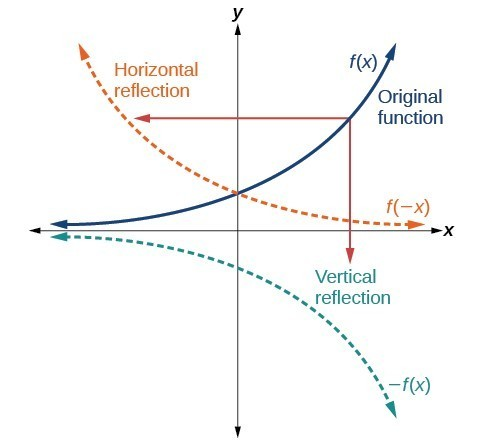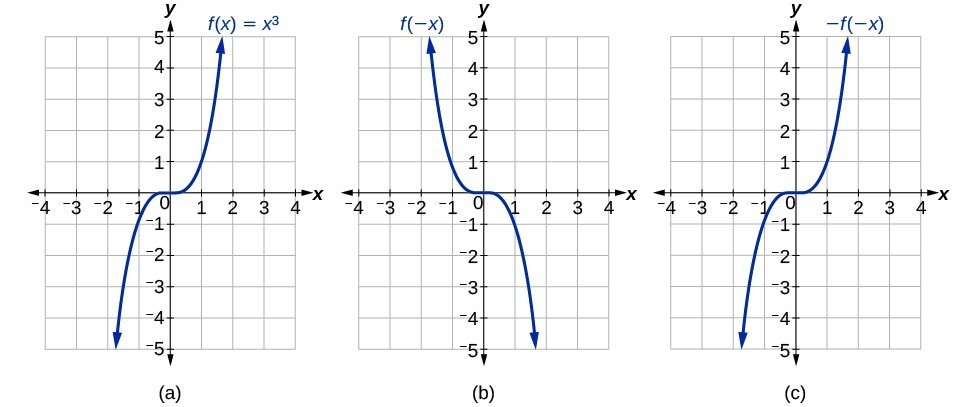## Reflections

### Learning Outcomes

• Graph functions using reflections about the $x$ -axis and the $y$ -axis.
• Determine whether a function is even, odd, or neither from its graph.

Another transformation that can be applied to a function is a reflection over the $x$– or $y$-axis. A vertical reflection reflects a graph vertically across the $x$-axis, while a horizontal reflection reflects a graph horizontally across the $y$-axis. The reflections are shown in Figure 9.Vertical and horizontal reflections of a function.

Notice that the vertical reflection produces a new graph that is a mirror image of the base or original graph about the $x$-axis. The horizontal reflection produces a new graph that is a mirror image of the base or original graph about the $y$-axis.

### A General Note: Reflections

Given a function $f\left(x\right)$, a new function $g\left(x\right)=-f\left(x\right)$ is a vertical reflection of the function $f\left(x\right)$, sometimes called a reflection about (or over, or through) the $x$-axis.

Given a function $f\left(x\right)$, a new function $g\left(x\right)=f\left(-x\right)$ is a horizontal reflection of the function $f\left(x\right)$, sometimes called a reflection about the $y$-axis.

### How To: Given a function, reflect the graph both vertically and horizontally.

1. Multiply all outputs by –1 for a vertical reflection. The new graph is a reflection of the original graph about the $x$-axis.
2. Multiply all inputs by –1 for a horizontal reflection. The new graph is a reflection of the original graph about the $y$-axis.

### Example: Reflecting a Graph Horizontally and Vertically

Reflect the graph of $s\left(t\right)=\sqrt{t}$  (a) vertically and (b) horizontally.

### Try It

Use an online graphing tool to reflect the graph of $f\left(x\right)=|x - 1|$ (a) vertically and (b) horizontally.

### Example: Reflecting a Tabular Function Horizontally and Vertically

A function $f\left(x\right)$ is given. Create a table for the functions below.

1. $g\left(x\right)=-f\left(x\right)$
2. $h\left(x\right)=f\left(-x\right)$
 $x$ 2 4 6 8 $f\left(x\right)$ 1 3 7 11

### Try It

 $x$ −2 0 2 4 $f\left(x\right)$ 5 10 15 20

Using the function $f\left(x\right)$ given in the table above, create a table for the functions below.

a. $g\left(x\right)=-f\left(x\right)$

b. $h\left(x\right)=f\left(-x\right)$

## Determine Whether a Functions is Even, Odd, or Neither

Some functions exhibit symmetry so that reflections result in the original graph. For example, horizontally reflecting the toolkit functions $f\left(x\right)={x}^{2}$ or $f\left(x\right)=|x|$ will result in the original graph. We say that these types of graphs are symmetric about the $y$-axis. Functions whose graphs are symmetric about the y-axis are called even functions.

If the graphs of $f\left(x\right)={x}^{3}$ or $f\left(x\right)=\dfrac{1}{x}$ were reflected over both axes, the result would be the original graph.(a) The cubic toolkit function (b) Horizontal reflection of the cubic toolkit function (c) Horizontal and vertical reflections reproduce the original cubic function.

We say that these graphs are symmetric about the origin. A function with a graph that is symmetric about the origin is called an odd function.

Note: A function can be neither even nor odd if it does not exhibit either symmetry. For example, $f\left(x\right)={2}^{x}$ is neither even nor odd. Also, the only function that is both even and odd is the constant function $f\left(x\right)=0$.

### A General Note: Even and Odd Functions

A function is called an even function if for every input $x$

$f\left(x\right)=f\left(-x\right)$

The graph of an even function is symmetric about the $y\text{-}$ axis.

A function is called an odd function if for every input $x$

$f\left(x\right)=-f\left(-x\right)$

The graph of an odd function is symmetric about the origin.

### How To: Given the formula for a function, determine if the function is even, odd, or neither.

1. Determine whether the function satisfies $f\left(x\right)=f\left(-x\right)$. If it does, it is even.
2. Determine whether the function satisfies $f\left(x\right)=-f\left(-x\right)$. If it does, it is odd.
3. If the function does not satisfy either rule, it is neither even nor odd.

### Example: Determining whether a Function Is Even, Odd, or Neither

Is the function $f\left(x\right)={x}^{3}+2x$ even, odd, or neither?

### Try It

Is the function $f\left(s\right)={s}^{4}+3{s}^{2}+7$ even, odd, or neither?

## Contribute!

Did you have an idea for improving this content? We’d love your input.